# Riemann-Roch theorem

(diff) ← Older revision | Latest revision (diff) | Newer revision → (diff)

A theorem expressing the Euler characteristicof a locally free sheaf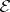on an algebraic or analytic varietyin terms of the characteristic Chern classes ofand(cf. Chern class). It can be used to calculate the dimension of the space of sections of(the Riemann–Roch problem).

The classical Riemann–Roch theorem relates to the case of non-singular algebraic curvesand states that for any divisoron,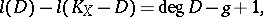(1)

whereis the dimension of the space of functions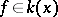for which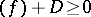,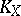is the canonical divisor andis the genus of. In the middle of the 19th century B. Riemann used analytic methods to obtain the inequality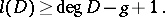The equality (1) was proved by E. Roch.

The Riemann–Roch theorem for curves is the one-dimensional case of the more general Riemann–Roch–Hirzebruch–Grothendieck theorem. Letbe a non-singular projective variety of dimension, and let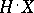be an appropriate cohomology theory: eitherare singular cohomology spaces when the basic field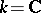, orwhere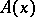is a Chow ring, oris the ring associated to the Grothendieck ring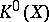(see , ). Letbe a locally free sheaf of rankon. Universal polynomials forwith rational coefficients,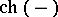and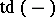, in the Chern classes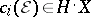ofare defined in the following way. The factorizationis examined for the Chern polynomial, where theare formal symbols. The exponential Chern character is defined by the formulaand, accordingly, the Todd class is defined as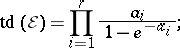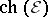and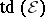are symmetric functions in the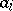and they can be written as polynomials in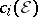.

The Riemann–Roch–Hirzebruch theorem: Ifis a non-singular projective variety or a compact complex variety of dimensionand ifis a vector bundle of rankon, thenwhere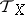is the tangent sheaf onand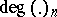denotes the component of degreein. This theorem was proved by F. Hirzebruch in the case of the ground field. Whenand the invertible sheaf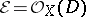, it leads to the equation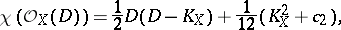whereis the second Chern class of the surfaceandis its canonical class. In particular, whenNoether's formula is obtained: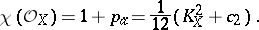For three-dimensional varietiesthe theorem leads to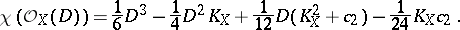In particular, when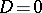,In 1957, A. Grothendieck generalized the Riemann–Roch–Hirzebruch theorem to the case of a morphism of non-singular varieties over an arbitrary algebraically closed field (see ). Let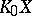andbe the Grothendieck groups of the coherent and locally free sheafs on, respectively (cf. Grothendieck group). The functoris a covariant functor from the category of schemes and proper morphisms into the category of Abelian groups. In this case, for a proper morphismthe morphism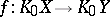is defined by the formula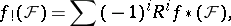where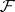is an arbitrary coherent sheaf onandis a covariant functor into the category of rings. For regular schemes with an ample sheaf, the groupsand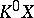coincide and are denoted by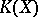. The Chern character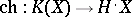is a homomorphism of rings;is also a covariant functor: The Gizin homomorphism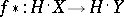is defined. When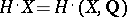, the homomorphism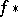is obtained fromfor homology spaces using Poincaré duality. The theorem as generalized by Grothendieck expresses the measure of deviation from commutativity of the homomorphisms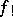and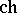.

The Riemann–Roch–Hirzebruch–Grothendieck theorem: Letbe a smooth projective morphism of non-singular projective varieties; then for any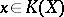the equation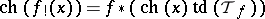inis true, where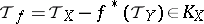(the relative tangent sheaf of the morphism).

Whenis a point, this theorem reduces to the Riemann–Roch–Hirzebruch theorem. There are generalizations (see , , ) whenis a Noetherian scheme with an ample invertible sheaf, whenis a projective morphism whose fibres are locally complete intersections, and also to the case of singular quasi-projective varieties.

Several versions of the Riemann–Roch theorem are closely connected with the index problem for elliptic operators (see Index formulas). For example, the Riemann–Roch–Hirzebruch theorem for compact complex varieties is a particular case of the Atiyah–Singer index theorem.

How to Cite This Entry:
Riemann-Roch theorem. Encyclopedia of Mathematics. URL: http://encyclopediaofmath.org/index.php?title=Riemann-Roch_theorem&oldid=15175
This article was adapted from an original article by Val.S. Kulikov (originator), which appeared in Encyclopedia of Mathematics - ISBN 1402006098. See original article